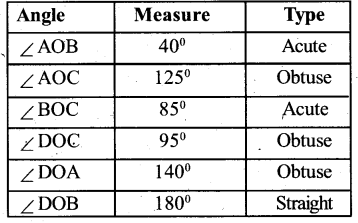# KSEEB Solutions for Class 6 Maths Chapter 5 Understanding Elementary Shapes Ex 5.4

Students can Download Chapter 5 Understanding Elementary Shapes Ex 5.4 Questions and Answers, Notes Pdf, KSEEB Solutions for Class 6 Maths helps you to revise the complete Karnataka State Board Syllabus and score more marks in your examinations.

## Karnataka State Syllabus Class 6 Maths Chapter 5 Understanding Elementary Shapes Ex 5.4

Question 1.
What is the measure of
(i) a right angle?
(ii) a straight angle?
Solution:
i) The measure of a right angle is 90°
ii) The measure of a straight angle is 180°

Question 2.
Say True or false:
a) The measure of an acute angle <90°
True

b) The measure of an obtuse angle <90° False c) The measure of reflex angle > 180°
True

d) The measure of one complete revolution = 360°
True

e) If m∠A = 53° and m∠B = 35°, then m∠A > m∠B.
TrueQuestion 3.
Write down the measures of Some acute angles Some obtuse angles (given at least two examples of each)
Solution:
a) 45°, 70°
b) 105°, 132°

Question 4.
Measure the angles given below using the protractor and write down the measure.
Solution: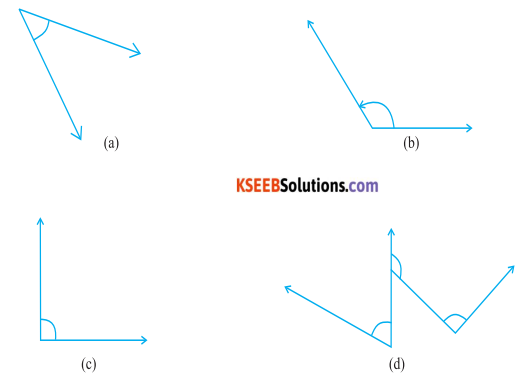Solution: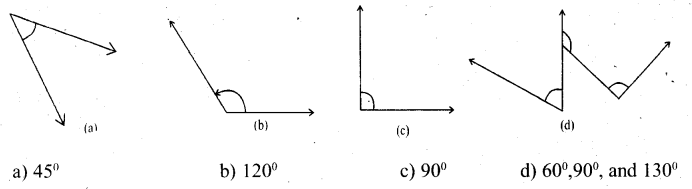Question 5.
Which angle has a large measure? First estimate and then measure.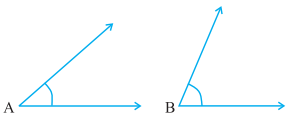Solution:
Measure of Angle A = 40°
Measure of Angle B = 68°
∠B has the greater measure than ∠AQuestion 6.
From these two angles Which has largest measure? Estimate and then confirm by measuring them.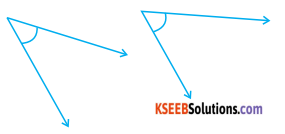Solution:
The measures of these angles are 45° and 55° Therefore the angle shown in 2nd figure is greater.

Question 7.
Fill in the blanks with acute, obtuse, right or straight:
a) An angle who se measure is greater than that of a right angle is Acute angle
b) An angle whose measure is greater than that of a right angle is obtuse
c) An angle Whose measure is the sum of the measures of two right angles is Straight angle
d) When the sum of the measure of two angles is that of a right angle, then each one of them is Acute
e) When the sum of the measures of two angles is that of a straight angle and it one of them is acute then the other should be obtuse angle

Question 8.
Find the measure of the angle shown in each figure. ( First estimate with your eyes and then find the actual measure with a protractor).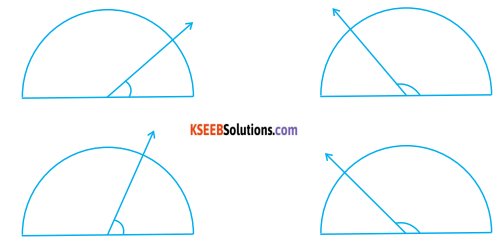Solution:
The measures of the angle shown in the above figure are 40°, 130°,65°, 135° respectively.Question 9.
Find the angle measure between the hands of the clock in each figure:
Solution: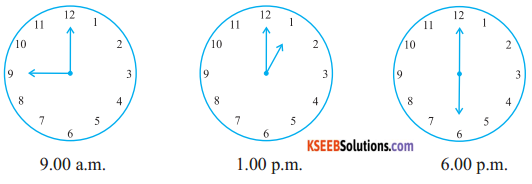Question 10.
Investigate
In the given figure, the angle measures 300. Look at the same figure through a magnifying glass. Does the angle becomes larger? Does the size of the angle change?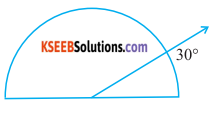Solution:
The measure of this angle will not changeQuestion 11.
Measure and classify each angle.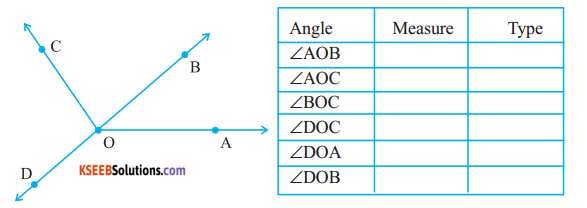Solution: